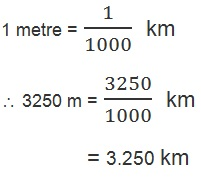Study Materials: ncert solutions

Our ncert solutions for Solutions 10. MOTION AND MEASUREMENT OF DISTANCES - Exercise (NCERT Text Book) | Class 6 Science - Toppers Study is the best material for English Medium students cbse board and other state boards students.

Solutions ⇒ Class 6th ⇒ Science ⇒ 10. MOTION AND MEASUREMENT OF DISTANCES

# Solutions 10. MOTION AND MEASUREMENT OF DISTANCES - Exercise (NCERT Text Book) | Class 6 Science - Toppers Study

Topper Study classes prepares ncert solutions on practical base problems and comes out with the best result that helps the students and teachers as well as tutors and so many ecademic coaching classes that they need in practical life. Our ncert solutions for Solutions 10. MOTION AND MEASUREMENT OF DISTANCES - Exercise (NCERT Text Book) | Class 6 Science - Toppers Study is the best material for English Medium students cbse board and other state boards students.

## Solutions 10. MOTION AND MEASUREMENT OF DISTANCES - Exercise (NCERT Text Book) | Class 6 Science - Toppers Study

CBSE board students who preparing for class 6 ncert solutions maths and Science solved exercise chapter 10. MOTION AND MEASUREMENT OF DISTANCES available and this helps in upcoming exams 2023-2024.

### You can Find Science solution Class 6 Chapter 10. MOTION AND MEASUREMENT OF DISTANCES

• All Chapter review quick revision notes for chapter 10. MOTION AND MEASUREMENT OF DISTANCES Class 6
• NCERT Solutions And Textual questions Answers Class 6 Science
• Extra NCERT Book questions Answers Class 6 Science
• Importatnt key points with additional Assignment and questions bank solved.

Chapter 10 Science class 6

### Exercise (NCERT Text Book) class 6 Science Chapter 10. MOTION AND MEASUREMENT OF DISTANCES

• Solutions 10. MOTION AND MEASUREMENT OF DISTANCES - Exercise (NCERT Text Book) | Class 6 Science - Toppers Study
• Class 6 Ncert Solutions
• Solution Chapter 10. MOTION AND MEASUREMENT OF DISTANCES Class 6
• Solutions Class 6
• Chapter 10. MOTION AND MEASUREMENT OF DISTANCES Exercise (NCERT Text Book) Class 6

## Solutions 10. MOTION AND MEASUREMENT OF DISTANCES - Exercise (NCERT Text Book) | Class 6 Science - Toppers Study

### Text book Exercise:

Q1. Give two examples each, of modes of transport used on land, water and air.

Ans: Two examples of each.

Land transport: bus and train.

Water transport: boat and   ship.

Air transport: Helicopter and aeroplane.

Q2. Fill in the blanks:
(i) One metre is ______________ cm.
(ii) Five kilometre is ______________ m.
(iii)Motion of a child on a swing is ______________.
(iv) Motion of the needle of a sewing machine is ______________.
(v) Motion of wheel of a bicycle is______________.

Ans:

(i) 100

(ii)  500

(iii) periodic motion

(iv) periodic motion

(v)  rotational motion

Q3. Why can a pace or a footstep not be used as a standard unit of length?

Ans: Standarrd unit of length must have a fixed numeric value while footstep does have so.

Q4. Arrange the following lengths in their increasing magnitude:
1 metre, 1 centimetre, 1 kilometre,1 millimetre.

Ans: 1 milimetre, 1 centimetre, 1 metre, 1 kilometre

Q5. The height of a person is 1.65 m. Express it into cm and mm.

Ans:

The height of a person = 1.65 m

In centimetre,

1 metre = 100 cm

1.65 m = 1.65 x 100 cm

= 165 cm

In milimetre,

1 centimetre = 10 milimetre

1 metre = 100 centimetre

Or        = 100 x 10 milimetre

= 1000 milimetre

1.65 metre = 1.65 x 1000 milimetre

= 1650 milimetre

Q6. The distance between Radha's home and her school is 3250 m. Express this distance into km.

Ans:Q7. While measuring the length of a knitting needle, the reading of the scale at one end is 3.0 cm and at the other end is 33.1 cm. What is the length of the needle?

Ans:

Reading of scale at one end = 3.0 cm

Reading of scale at other end = 33.1 cm

Distance between two end = 33.1 - 3.0 cm

= 30.1 cm

Q8. Write the similarities and differences between the motion of a bicycle and a
ceiling fan that has been switched on.

Ans:

Similarities: the motion of a bicycle and a celing fan both have circular motion.

Differences: The bicycle moves on straight line but ceiling fan does not move so.

Q9. Why could you not use an elastic measuring tape to measure distance? What would be some of the problems you would meet in telling someone about a distance you measured with an elastic tape?

Ans:

Q10. Give two examples of periodic motion.

Ans:

(i) The motion of child on a swing.

(ii) moving here and there the branches of tree.

##### Other Pages of this Chapter: 10. MOTION AND MEASUREMENT OF DISTANCES

Important Study materials for classes 06, 07, 08,09,10, 11 and 12. Like CBSE Notes, Notes for Science, Notes for maths, Notes for Social Science, Notes for Accountancy, Notes for Economics, Notes for political Science, Noes for History, Notes For Bussiness Study, Physical Educations, Sample Papers, Test Papers, Mock Test Papers, Support Materials and Books.Mathematics Class - 11th

NCERT Maths book for CBSE Students.

books

## Study Materials List:

##### Solutions ⇒ Class 6th ⇒ Science
1. FOOD: WHERE DOES IT COME FROM
2. COMPONENTS OF FOOD
3. FIBRE TO FABRIC
4. SORTING MATERIAL INTO GROUPS
5. SEPARATION OF SUBSTANCES
6. CHANGES AROUND US
7. GETTING TO KNOW PLANTS
8. BODY MOVEMENTS
9. THE LIVING ORGANISMS AND THEIR SURROUNDINGS
10. MOTION AND MEASUREMENT OF DISTANCES